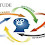## This project is to implement a parameterized multiplier using carry-look-ahead adders in Verilog. The Verilog code for the multiplier is provided.

Users can change the number of bits of the multiplier by modifying the predefined parameters. The parameters such as MULTICAND_WID and MULTIPLIER_WID are to define the number of bits of the multiplicand and multiplier, and when we want to change the number of bits, just change these parameters and re-synthesize or simulate.````timescale 1ns/1ps
`define DELAY #10
// fpga4student.com FPGA projects, Verilog projects, VHDL projects
module cpu_wb_cla_adder (in1, in2, carry_in, sum, carry_out);
parameter DATA_WID = 32;

input [DATA_WID - 1:0] in1;
input [DATA_WID - 1:0] in2;
input carry_in;
output [DATA_WID - 1:0] sum;
output carry_out;

//assign {carry_out, sum} = in1 + in2 + carry_in;

wire [DATA_WID - 1:0] gen;
wire [DATA_WID - 1:0] pro;
wire [DATA_WID:0] carry_tmp;

genvar j, i;
generate
//assume carry_tmp in is zero
assign carry_tmp = carry_in;

//carry generator
for(j = 0; j < DATA_WID; j = j + 1) begin: carry_generator
assign gen[j] = in1[j] & in2[j];
assign pro[j] = in1[j] | in2[j];
assign carry_tmp[j+1] = gen[j] | pro[j] & carry_tmp[j];
end

//carry out
assign carry_out = carry_tmp[DATA_WID];

//calculate sum
//assign sum = in1 ^ in2 ^ carry_in;
for(i = 0; i < DATA_WID; i = i+1) begin: sum_without_carry
assign sum[i] = in1[i] ^ in2[i] ^ carry_tmp[i];
end
endgenerate
endmodule```

``` module cla_adder_tb();
parameter DATA_WID = 16;
// fpga4student.com FPGA projects, Verilog projects, VHDL projects
reg carry_in; // To cla1 of cla_adder.v
reg [DATA_WID-1:0] in1; // To cla1 of cla_adder.v
reg [DATA_WID-1:0] in2; // To cla1 of cla_adder.v

// fpga4student.com FPGA projects, Verilog projects, VHDL projects // /*AUTOWIRE*/
wire carry_out; // From cla1 of cla_adder.v
wire [DATA_WID-1:0] sum; // From cla1 of cla_adder.v

// fpga4student.com FPGA projects, Verilog projects, VHDL projects //
//  Outputs
.sum (sum[DATA_WID-1:0]),
.carry_out (carry_out),
// // Inputs
.in1 (in1[DATA_WID-1:0]),
.in2 (in2[DATA_WID-1:0]),
.carry_in (carry_in));

initial begin
in1 = 16'd0;
in2 = 16'd0;
carry_in = 1'b0;
end
// fpga4student.com FPGA projects, Verilog projects, VHDL projects
initial begin
#(`DELAY)
#(`DELAY)  in1 = 16'd10;
#(`DELAY)  in1 = 16'd20;
#(`DELAY)  in2 = 16'd10;
#(`DELAY)  in2 = 16'd20;
#(`DELAY)  in2 = 16'd0;
#(`DELAY*3)  in1 = 16'hFFFF; in2 = 16'hFFFF;
#(`DELAY*3)  in1 = 16'h7FFF; in2 = 16'hFFFF;
#(`DELAY*3)  in1 = 16'hBFFF; in2 = 16'hFFFF;
end
endmodule
```
Finally, the Verilog and testbench code for the parameterized carry look-ahead multiplier.

````timescale 1ns/1ps
`define DELAY 10
// fpga4student.com FPGA projects, Verilog projects, VHDL projects
module cpu_wb_cla_multiplier (multicand, multiplier, product);
parameter MULTICAND_WID = 32;
parameter MULTIPLIER_WID = 32;

input [MULTICAND_WID-1:0] multicand;
input [MULTIPLIER_WID-1:0] multiplier;
output [(MULTICAND_WID + MULTIPLIER_WID - 1):0] product;

wire [MULTICAND_WID - 1:0] multicand_tmp [MULTIPLIER_WID-1:0];
wire [MULTICAND_WID - 1:0] product_tmp [MULTIPLIER_WID-1:0];
wire [MULTIPLIER_WID -1:0] carry_tmp;
// fpga4student.com FPGA projects, Verilog projects, VHDL projects genvar i, j;
generate
//initialize values
for(j = 0; j < MULTIPLIER_WID; j = j + 1) begin: for_loop_j
assign multicand_tmp[j] =  multicand & {MULTICAND_WID{multiplier[j]}};
end

assign product_tmp = multicand_tmp;
assign carry_tmp = 1'b0;
assign product = product_tmp;
// fpga4student.com FPGA projects, Verilog projects, VHDL projects
for(i = 1; i < MULTIPLIER_WID; i = i + 1) begin: for_loop_i
// Outputs
.sum(product_tmp[i]),
.carry_out(carry_tmp[i]),
// Inputs
.carry_in(1'b0),
.in1(multicand_tmp[i]),
.in2({carry_tmp[i-1],product_tmp[i-1][31-:31]}));
assign product[i] = product_tmp[i];
end //end for loop
assign product[(MULTIPLIER_WID+MULTIPLIER_WID-1):MULTIPLIER_WID] = {carry_tmp[MULTIPLIER_WID-1],product_tmp[MULTIPLIER_WID-1][31-:31]};
endgenerate
endmodule
```

#### Verilog testbench code for the Multiplier:

```// Verilog project: Verilog code for multiplier using carry look ahead adder
// fpga4student.com FPGA projects, Verilog projects, VHDL projects
module cla_multiplier_tb();
parameter MULTICAND_WID = 32;
parameter MULTIPLIER_WID = 32;
// fpga4student.com FPGA projects, Verilog projects, VHDL projects // /*AUTOREGINPUT*/
// Beginning of automatic reg inputs (for undeclared instantiated-module inputs)
reg [MULTICAND_WID-1:0] multicand; // To mul1 of cla_multiplier.v
reg [MULTIPLIER_WID-1:0]multiplier; // To mul1 of cla_multiplier.v
// End of automatics

/*AUTOWIRE*/
// Beginning of automatic wires (for undeclared instantiated-module outputs)
wire [(MULTICAND_WID+MULTIPLIER_WID-1):0]product;// From mul1 of cla_multiplier.v
// End of automatics

cpu_wb_cla_multiplier mul1(/*AUTOINST*/
// // Outputs
.product (product[(MULTICAND_WID+MULTIPLIER_WID-1):0]),
// // Inputs
.multicand (multicand[MULTICAND_WID-1:0]),
.multiplier (multiplier[MULTIPLIER_WID-1:0]));

//initial begin
// multicand = 16'd0;
// multiplier = 8'd0;
// end
// fpga4student.com FPGA projects, Verilog projects, VHDL projects  integer i;
initial begin
for (i = 0; i < 30; i = i + 1) begin: W
#(`DELAY) multicand = multicand + 1; multiplier = multiplier + 1;
end

#(`DELAY) //correct
multicand = 32'h00007FFF;
multiplier = 32'h0000007F;

#(`DELAY) //correct
multicand = 32'h00008000;
multiplier = 32'h000000F0;

#(`DELAY) //faila
multicand = 32'h00008FF0;
multiplier = 32'h000000F0;

#(`DELAY) //correct
multicand = 32'h00007FF0;
multiplier = 32'h000000F7;

#(`DELAY) //correct
multicand = 32'h0000FFFF;
multiplier = 32'h000000FF;
end
// fpga4student.com FPGA projects, Verilog projects, VHDL projects  endmodule
```

#### Simulation results for the Verilog multiplier:1.Thanks. Please keep updating the blog: http://www.fpga4student.com/

2.Hello,
Do you have code for divider in Verilog ? Thanks.

3.Verilog code for divider

4.Hi. Do you have code for booth encoded multiplier ?

5.No. But you can refer to this as a reference:
http://www.fpga4student.com/2016/11/matrix-multiplier-core-design.html

6.1.Check the shift/add multiplier in Verilog below:
http://www.fpga4student.com/2016/11/verilog-code-for-4x4-multiplier-using.html

7.i get the error :
Macro `DELAY is undefined.
while compiling....

1.Include this `define DELAY #10 before every module.

8.i am new to verilog and i want to understand the algorithm and logic used in this code... can you help me?

1.These are normal carry look ahead adder and multiplier architectures. You can easily find the lecture on Google pdf.

2.I dont understand how the shifting operation is done in this algorithm because thats how you multiply normally...i searched a lot in google..can you give me some reference to where i can look into the algorithm used in this code

3.It is like when you perform the multiplication in math. You multiply and add. That's how it works in this code.

9.Please share the reference to this algorithm used for multiplication

10.verilog code for carry look ahead adder is showing successfully syntax is correct but simulation is not displaying. Why?